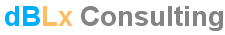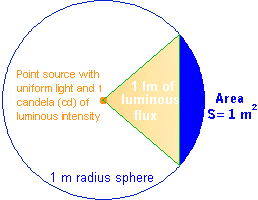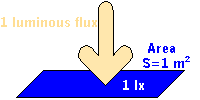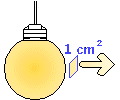Acoustics, Noise Control, Audio Systems and Lighting

Lighting Definitions

Luminous Flux
Luminous flux is the energy quantity of the light emitted per second in all directions. The unit of luminous flux is lumen (lm). One lumen is the luminous flux of the uniform point light source that has luminous intensity of 1 candela and is contained in one unit of spatial angle (or 1 steradian).

Steradian is the spatial angle that limits the surface area of the sphere equal to the square of the radius. This concept is shown in the figure for 1 m radius of the sphere. Since the area of sphere is 4.Π.r² then the luminous flux of the point light source is 4.Π lumens.Luminous Intensity
Luminous intensity is the ability to emit light into a given direction, or it is the luminous flux that is radiated by the light source in a given direction within the unit of the spatial angle. If the point light source emits Ø lumens into a small spatial angle ß, the luminous intensity is I=Ø/ß.

The unit of luminous intensity is candela. There is a standard that details the candela definition. This includes the standard light source and the physical conditions of the measurement.

Illuminance (Illumination)
This definition determines the amount of light that covers a surface. If Ø is the luminous flux and S is the area of the given surface, then the illuminance E is determined by E=Ø/S.

The unit of illumination in SI system is lx, and in foot-pound system it is foot-candle. One lx is the illuminance of 1 m² surface area uniformly lighted by 1 lm of luminous flux. The following drawing explains this definition. One foot-candle is 10.76 lux.Luminance
Luminance L is the luminous intensity emitted by the surface area of 1 cm² (or 1 m²) of the light source. Mathematically it is L=I/S where I is the luminous intensity and S is the area of the source surface perpendicular to the given direction.

The unit of luminance is cd/m² or cd/cm² (in some applications lm/cm² or Lambert can be used). The following figure shows the concept.The luminance value indicates glare and discomfort when we look at the lighting source. These values are very high for the sun or a small lamp and are low for large lighting fixtures.